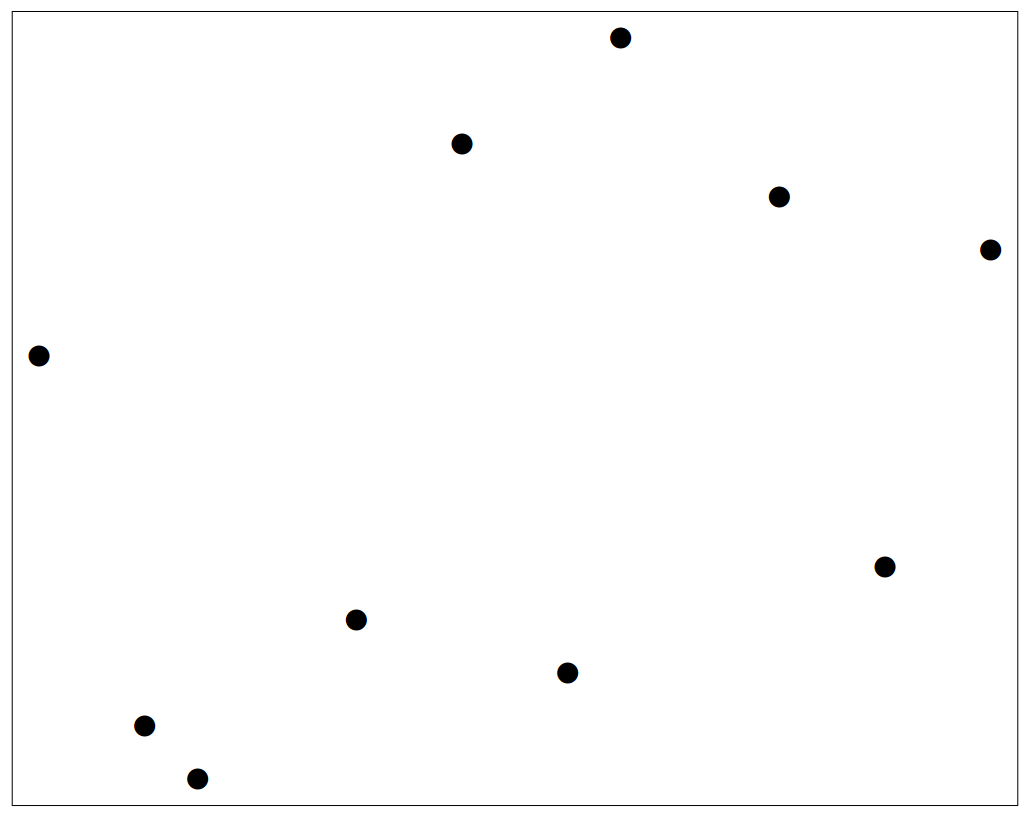CDQ分治 | Kyons'Blog

## 不得不说

本来标题想写分治,但是想了想发现自己分治能说的不多,主要的内容就是$CDQ$分治.便取了这个标题.

## 预备知识

• 关于什么是分治
分治，字面上的解释是“分而治之”，就是把一个复杂的问题分成两个或更多的相同或相似的子问题，再把子问题分成更小的子问题……直到最后子问题可以简单的直接求解，原问题的解即子问题的解的合并。在计算机科学中，分治法就是运用分治思想的一种很重要的算法。分治法是很多高效算法的基础，如排序算法（快速排序，归并排序），傅立叶变换（快速傅立叶变换）等等。
• 一般步骤
1. 划分步：把输入的问题划分为$k$个子问题，并尽量使这$k$个子问题的规模大致相同。
2. 治理步：当问题的规模大于某个预定的阈值$n_0$时，治理步由$k$个递归调用组成。
3. 组合步：组合步把各个子问题的解组合起来，它对分治算法的实际性能至关重要，算法的有效性很大地依赖于组合步的实现。
• 时间复杂度
• 直观估计
• 分治由以上三部分构成，整体时间复杂度则由这三部分的时间复杂度之和构成.
• 由于递归，最终的子问题变得极为简单，以至于其时间复杂度在整个分治策略上的比重微乎其微.
• 经典例题
• 题目简析:
• 问多少种子序列,子序列中的字母不同.
• 列如$baa$,包括:$b,a,a,$两个不同位置$a$的$ba$,总计$5$个,$baa$排除是因为$a$是重复的.
• 解法
• 先将每个字母的个数统计下来,然后分治计算,一个字母的时候,答案是该字母出现的次数.
• 只有两个字母的时候,如$ab$,包含的排列有$a,b,ab$,相当于’$a$的个数,$b$的个数,$a$和$b$组合个数’的加和,而$a$和$b$组合个数,则是$a$的个数$\times b$的个数
• 同理可得,$ans$即为$ansL+ansR+ansL\times ansR$.

## CDQ分治

• 优势在于可以顶替复杂的高级数据结构，而且常数比较小
• 缺点在于必须离线操作

• 首先,分治问题2333
• 分治后的答案,不仅单单考虑子问题${L,mid}$和子问题${mid+1,R}$.
• 还需要考虑子问题${L,mid}$对子问题${mid+1,R}$的影响$/$联系产生的答案.

• 二维偏序问题
• 给定一个二元组${x,y}$,要求问有多少对${x_i,y_i},{x_j,y_j}$满足$x_i>x_j$&&$y_i>y_j$
• 解法为:
• 先将二元组按照$x$的大小排列.
• 分治后,我们分别知道${L,mid}$区间和${mid+1,R}$区间内的解
• 再计算跨过$mid$的两对点,对${L,mid}$和${mid+1,R}$中的二元组按照$y$的大小排序
• 由于先前分组便已经对$x$进行排序,所以,只需要二分便可以求得左区间相对于右区间的点的个数.
• 三维偏序问题
• 和二维偏序问题类似,但有一定不同
• 给定一个三元组${x,y,z}$,要求问有多少对${x_i,y_i,z_i},{x_j,y_j,z_j}$满足$x_i>x_j$&&$y_i>y_j$&&$z_i>z_j$
• 解法为:
• 先将三元组按照$x$的大小排列.
• 分治后,我们分别知道${L,mid}$区间和${mid+1,R}$区间内的解
• 再计算跨过$mid$的两对点,对${L,mid}$和${mid+1,R}$中的三元组按照$y$的大小排序
• 由于先前分组便已经对$x$进行排序,所以,只需要二分便可以求得满足$y$条件的点.
• 再建立一个权值树状数组$/$线段树,再将上面符合的${x,y,z}$对应中满足不等式的$z$的点求出.

## 例题:稻草人

### 问题简述

给定$n$个稻草人（横纵坐标是不大于$10^9$的非负整数且两两$x,y$都不相同）

1. 边平行于横、纵轴
2. 左下角、右上角都是给定的稻草人
3. 内部不包含其它稻草人

### 解析

$Step\ 1.{\ }$
首先考虑,如果无视第三条,那么,该题变为了什么?
给定二元组集合,$A={x,y}$,那么对于任何${x_i,y_i}$,${x_j,y_j}$,问有多少对点满足:$y_j>y_i$&&$x_j>x_i$
对于这样的一个式子,我们很容易得到两种解法,第一种:树状数组/万能的线段树.第二种:$cdq$分治.当然,本蒟蒻选择第二种.(为什么?树状数组咱还没学,线段树不会使)
然后呢,$cdq$分治的标准操作为?

$Step\ 2.{\ }$
于是,我们加上条件三.
如何处理两边点之间的关系?
对于左半边,我们假设右半边的点都是符合条件的.

1. 我们可以知道,如果左半边的一个点$A$是符合条件的,那么答案$ans+=$右半边对于$A$符合条件的点.
2. 我们在左半边加入$B$点,若$B$点的存在是与$A$点不冲突的,那么答案$ans+=$右半边对于$B$符合条件的点.
3. 若,$B$点与$A$点的存在有矛盾,即不符合条件三,列如$x_b<x_a,y_b>y_a$这种情况,我们应:将$A$点对应的值删去,加入右半边对于$B$符合条件的点

$Step\ 3.{\ }$
结合$Step\ 1$和$Step\ 2$.我们已知的有:
假设我们是对$x$按照从小到大排序的,即对点按照$x$离散化后.

1. 我们知道,左边的$x$必定小于右边的$x$.
2. 我们要按照y的大小对左右分别排序.
3. 对于右边,我们要维护一个按照$x$单调递增的单调栈.(即按照$y$递减)
4. 对于左边,我们要维护一个按照$x$单调递减的单调栈.(即按照$y$递增)
5. 每次左边新增一个$i$点,我们都要让答案$ans+=$右边维护的单调栈长度.答案$ans-=$右边比$y_i$小的点.
6. 我们知道单调栈是单调的….嗯,所以对于寻点,我们可以二分查找.

分析结束,综上,细节请参阅分治的代码来理解,手动模拟一遍就非常明了了.

输入
10
2 1
3 0
6 3
10 2
16 4
0 8
8 12
11 14
14 11
18 10
输出
15## 代码

//删除Valine核心代码库外链调用 //调用刚下载的本地文件以加速加载速度 //这里改为从本地加载